Function Repository Resource:

# PedalSurface

Compute a pedal surface

Contributed by: Wolfram Staff (original content by Alfred Gray)
 ResourceFunction["PedalSurface"][s,p,{u,v}] computes the pedal surface with respect to the point p of the surface s parameterized by variables {u,v}.

## Details and Options

The pedal of a surface s is the locus of the intersections of perpendicular lines from a fixed point p to the tangent planes of s.
Pedal surface is defined as the surface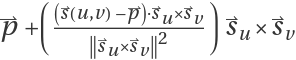.
The pedal surface is the 3D analog of a pedal curve.

## Examples

### Basic Examples (3)

Get the equation of an ellipsoid surface:

 In:=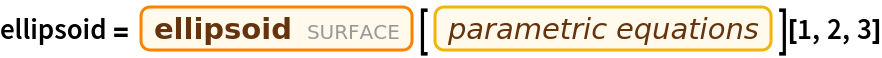Out=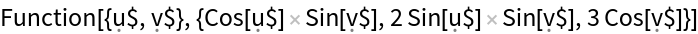Compute the pedal surface of the ellipsoid:

 In:=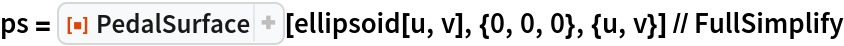Out=Plot the pedal surface; it is a particular case of Fresnel’s elasticity surface:

 In:=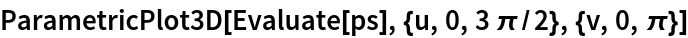Out=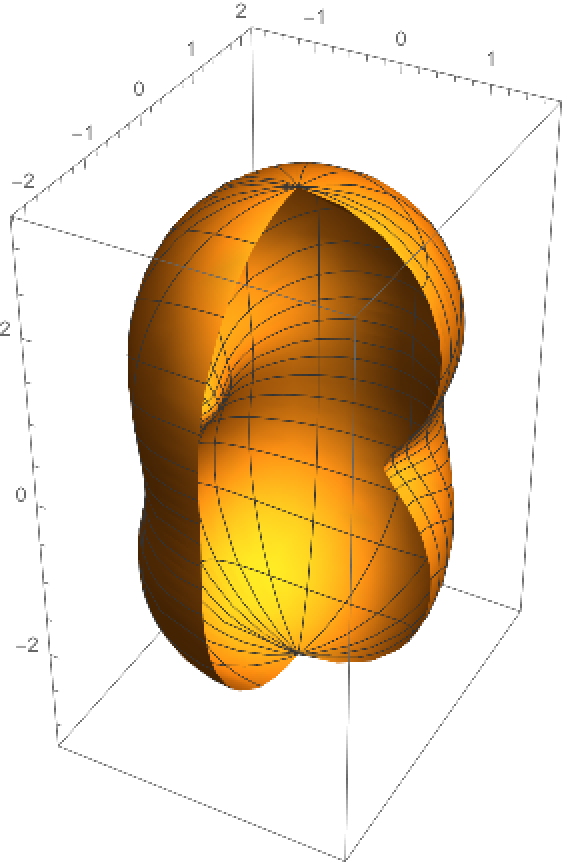### Scope (1)

The following Manipulate shows how the pedal surface is constructed. Note that the tangent plane at a point of the ellipsoid is perpendicular to the red line:

 In:=In:=Out=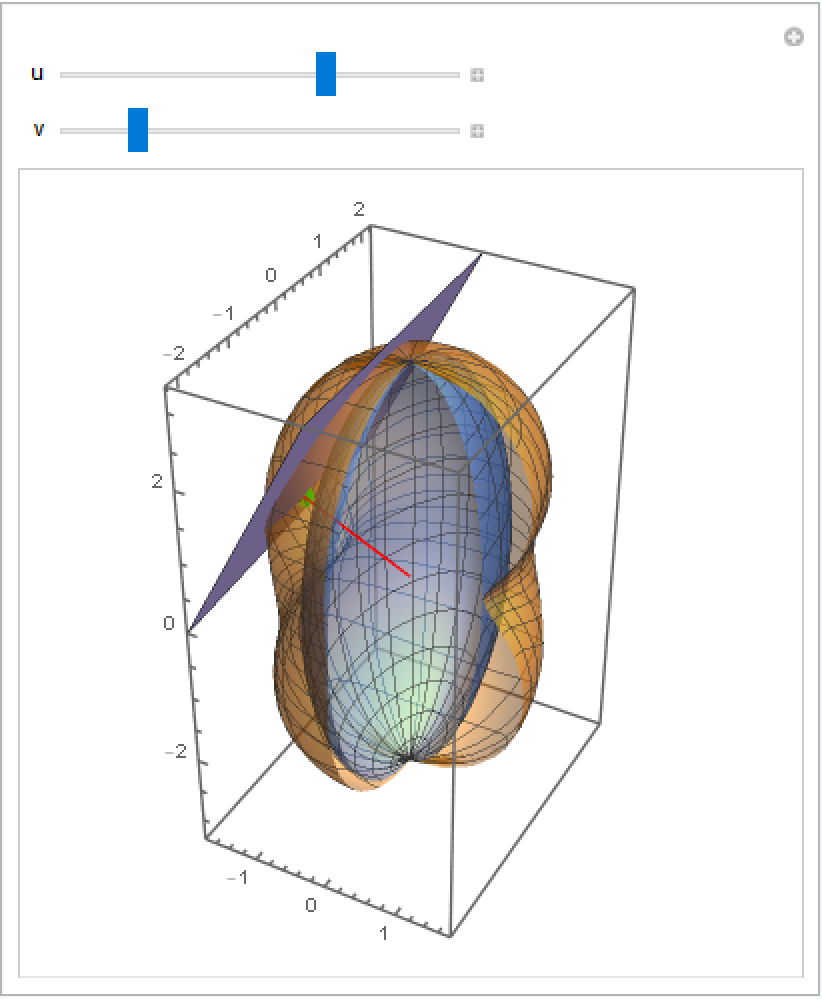### Properties and Relations (3)

Get the equation for an ellipse:

 In:=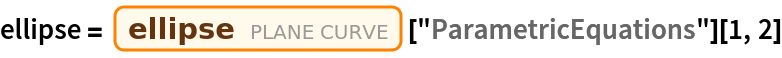Out=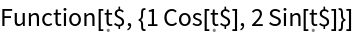Compute the pedal curve of the ellipse:

 In:=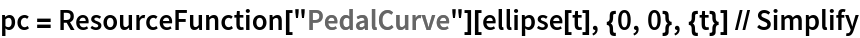Out=Plot the pedal curve; the contour is like the 3D case:

 In:=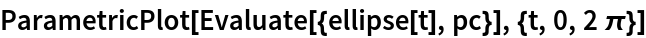Out=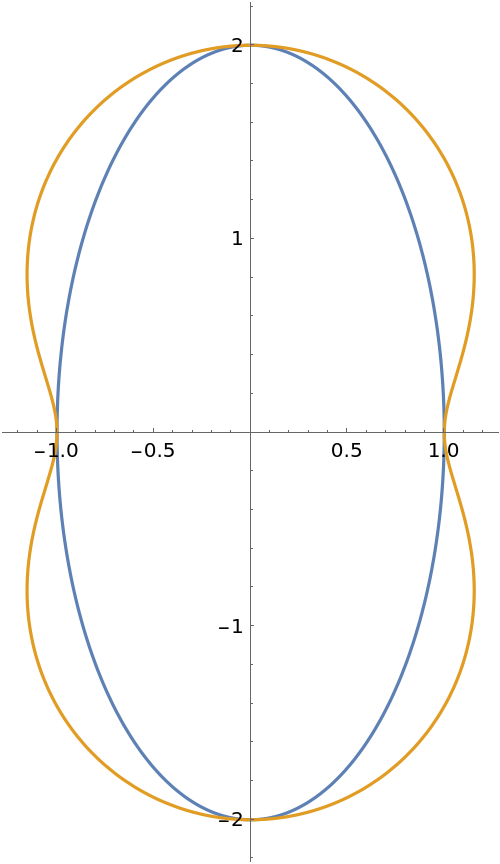Enrique Zeleny

## Version History

• 1.0.0 – 10 April 2020